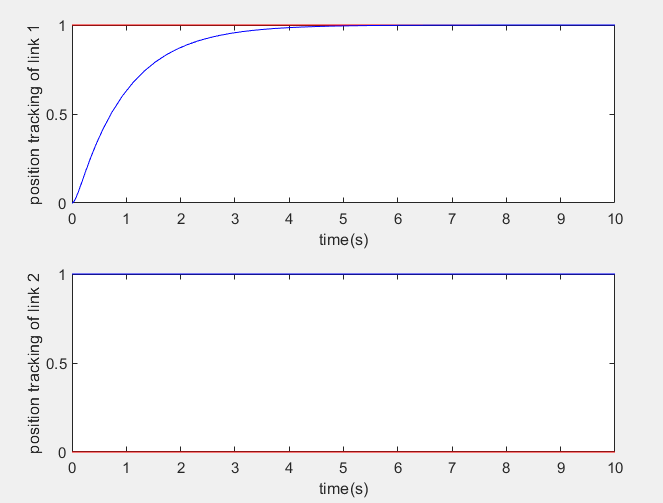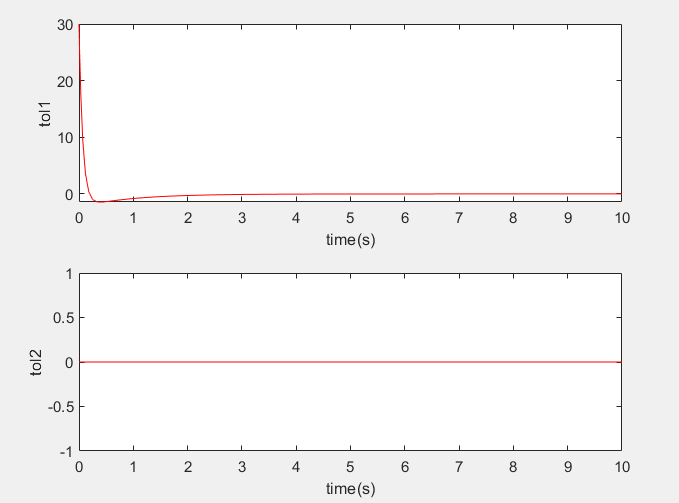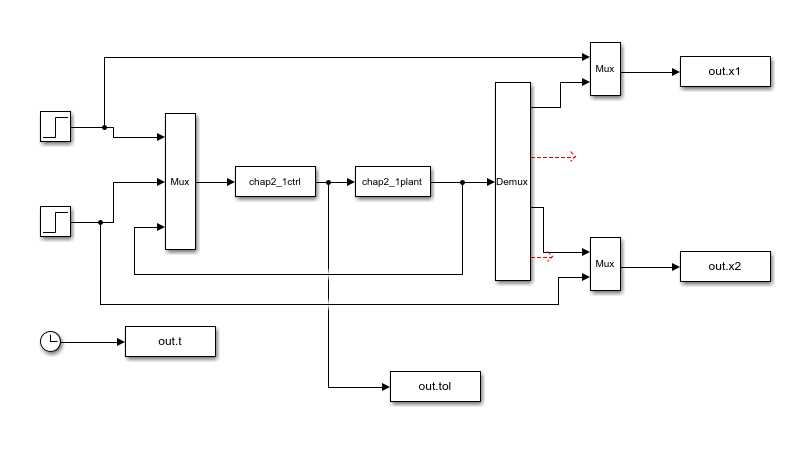# 五、机器人PID控制

## 5.1  机器人动力学模型及其结构特性

考虑一个N关节机器人，其动态性能可由二阶非线性微分方程描述：式中为关节角位移量， 为机器人的惯性矩阵，表示离心力和哥氏力，为重力项，表示摩擦力矩，为控制力矩，为外加扰动。

### 5.2.2  S函数使用步骤

一般而言，S函数的使用步骤如下：

### 5.2.3  S函数的基本功能及重要参数设定

（1）S函数功能模块，各种功能模块完成不同的任务，这些功能模块（函数）称为仿真例程或回调函数（call-back functions），包括初始化（initialization）、导数（mdlDerivative）、输出（mdlOutput）等。

（2）NumConStates表示S函数描述的模块中连续状态的个数。

（3）NumDiscStates表示离散状态的个数。

（4）NumOutputs和NumInputs分别表示模块输入和输出的个数。

（5）直接馈通（dirFeedthrough）为输入信号是否在输出端出现的标识，取值为0或1。

（6）NumSampleTimes为模块采用周期的个数，S函数支持多采样周期的系统。

除了sys外，还应设置系统的初始状态变量、说明变量和采样周期变量。变量为双列矩阵，其中每一行对应一个采样周期。对连续系统和单个采样周期的系统来说，该变量为，为采样周期，表示继承输入信号的采样周期；为偏移量，一般取为0。对连续系统来说，取为。

## 5.3  机器人独立PD控制

### 5.3.1  控制律设计

当忽略重力和外加干扰时，采用独立的PD控制，能满足机器人定点控制的要求。

设关节机械手方程为：

（5.1）

独立的PD控制律为：

（5.2）

取跟踪误差为，采用定点控制时，为常值，则

此时，机器人方程为：

（5.3）

取Lyapunov（李雅普诺夫）函数为：

### 5.3.2  收敛性分析

由于是半负定的，且为正定，则当时，有，从而。代入方程（5.3），有，再由的可逆性知。由LaSalle定理知，是受控机器人全局渐进稳定的平衡点，即从任意初始条件出发，均有

### 5.3.3  仿真实例

针对被控对象式（5.1），选二关节机器人系统（不考虑重力、摩擦力和干扰），其动力学模型为：

取，

位置指令为，在控制器式（5.2）中，取，仿真结果如图5.1和图5.2所示。图5.1  双力臂的阶跃响应图5.2  独立PD控制的控制输入

仿真中，当改变参数时，只要满足，都能获得比较好的仿真结果。完全不受外力没有任何干扰的机器人系统是不存在的，独立的PD控制只能作为基础来参考分析，但对它的分析是有意义的。

### 仿真程序function[sys,x0,str,ts] = chap2_1ctrl(t,x,u,flag)
%主函数
%主函数包括四个输出：
%                    sys数组包含某个子函数返回的值
%                    x0为所有状态的初始化变量
%                    str是保留参数，总是一个空矩阵
%                    ts返回系统采样时间
%函数的四个输入分别为采样时间t、状态x、输入u和仿真流程控制状态标志变量flag
%输入参数后面还可以接续一系列的附带参数simStateCompliance
switch flag
case 0
[sys,x0,str,ts] = mdlInitializeSizes;
case 3
sys = mdlOutputs(t,x,u);
case{2,4,9}
sys = [];
otherwise
error(['Unhandled flag = ',num2str(flag)]);
end

function[sys,x0,str,ts] = mdlInitializeSizes
%初始化回调子函数
%提供状态、输入、输出、采样时间数目和初始状态变量的值
%初始化阶段，标识变量flag首先被置为0，S-function首次被调用时
%该子函数首先被调用，且为S-function模块提供下面信息
%该子函数必须存在
sizes = simsizes;                 %用于设置参数的结构体用simsizes来生成
sizes.NumOutputs      = 2;        %模块输出变量的个数
sizes.NumInputs       = 6;        %模块输入变量的个数
sizes.DirFeedthrough  = 1;        %模块是否存在直接贯通，1存在，0不存在
sizes.NumSampleTimes  = 1;        %模块的采样时间个数，至少是一个
sys = simsizes(sizes);            %返回size数据结构所包含的信息
x0 = [];
str = [];
ts = [0 0];

function sys = mdlOutputs(t,x,u)
%计算输出回调函数
%给定t，x，u计算输出，可以在此描述系统的输出方程
%该子函数必须存在
R1 = u(1);dr1 = 0;                %u（1）是qd（1）
R2 = u(2);dr2 = 0;                %u（2）是qd（1）

x(1) = u(3);                      %u（3）是q（1）
x(2) = u(4);                      %u（4）是dq（1）(就是给q（1）求导)
x(3) = u(5);                      %u（5）是q（2）
x(4) = u(6);                      %u（6）是dq（2）(就是给q（2）求导)

e1 = R1 - x(1);
e2 = R2 - x(3);
e = [e1,e2];                      %跟踪误差e=qd-q

de1 = dr1 - x(2);                 %x（2）是dq（1）(就是给q（1）求导)
de2 = dr2 - x(4);                 %x（4）是dq（2）(就是给q（2）求导)
de = [de1;de2];                   %跟踪误差的导数de=d(qd-q)

Kp = [30 0;0 30];                 %Kp是P参数
Kd = [30 0;0 30];                 %Kd是D参数

tol = Kp.*e+Kd.*de;               %独立的PD控制律

sys(1) = tol(1);
sys(2) = tol(2);

%缺省的其他函数如下
%%%%%%%%%%%%%%%%%%%%%%%%%%%%%%%%%%%%%%%%%%%%%%%%%%%%%%%%%%%%%%%%%%%%%%%%%%%%%%%
%                                                                             %
%function sys = mdlUpdate(t,x,u)                                              %
%状态更新回调子函数                                                             %
%给定t、x、u计算离散状态的更新                                                  %
%每个仿真步内必然调用该子函数，不论是否有意义                                     %
%除了在此描述系统的离散状态方程外，还可以在此添加其他每个仿真步内都必须执行的代码    %
%%%%%%%%%%%%%%%%%%%%%%%%%%%%%%%%%%%%%%%%%%%%%%%%%%%%%%%%%%%%%%%%%%%%%%%%%%%%%%%

%%%%%%%%%%%%%%%%%%%%%%%%%%%%%%%%%%%%%%%%%%%%%%%%%%%%%%%%%%%%%%%%%%%%%%%%%%%%%%%
%                                                                             %
%function sys = mdlGetTimeOfNextVarHit(t,x,u)                                 %
%计算下一个采样时间                                                             %
%仅在系统是变采样时间系统时调用                                                  %
%%%%%%%%%%%%%%%%%%%%%%%%%%%%%%%%%%%%%%%%%%%%%%%%%%%%%%%%%%%%%%%%%%%%%%%%%%%%%%%

%%%%%%%%%%%%%%%%%%%%%%%%%%%%%%%%%%%%%%%%%%%%%%%%%%%%%%%%%%%%%%%%%%%%%%%%%%%%%%%
%function sys = mdlTerminate(t,x,u)                                           %
%仿真结束时要调用的回调函数                                                     %
%在仿真结束时，可以在此完成仿真结束所需的必要工作                                 %
%%%%%%%%%%%%%%%%%%%%%%%%%%%%%%%%%%%%%%%%%%%%%%%%%%%%%%%%%%%%%%%%%%%%%%%%%%%%%%%



%S-function for continuous state equation
function[sys,x0,str,ts] = chap2_1plant(t,x,u,flag)
switch flag
%Initialization
case 0
[sys,x0,str,ts] = mdlInitialSizes;
case 1
sys = mdlDerivatives(t,x,u);   %此时要计算连续状态的微分
%Outputs
case 3
sys = mdlOutputs(t,x,u);       %此时要计算输出
%Unhandled flags
case{2,4,9}
sys = [];
%Unexpected flags
otherwise
error(['Unhandled flag = ',num2str(flag)]);
end
%mdlInitialSizes
function[sys, x0,str,ts] = mdlInitialSizes
global p g
sizes = simsizes;                    %用于设置参数的结构体用simsizes来生成
sizes.NumContStates     = 4;         %模块连续状态变量的个数，缺省为0
sizes.NumDiscStates     = 0;         %模块离散状态变量的个数，缺省为0
sizes.NumOutputs        = 4;         %模块输出变量的个数，缺省为0
sizes.NumInputs         = 2;         %模块输入变量的个数，缺省为0
sizes.DirFeedthrough    = 0;         %模块是否存在直接贯通，1存在，0不存在
sizes.NumSampleTimes    = 0;         %模块的采样时间个数，至少是一个
sys = simsizes(sizes);               %设置完后赋给sys输出，返回size数据结构所包含的信息
x0 = [0 0 0 0];                      %状态变量设置为空，表示没有状态变量
str = [];                            %保留参数，置[]就可以，没什么用
ts = [];                             %采样周期，设为0表示是连续系统

p = [2.9 0.76 0.87 3.04 0.87];
g = 9.8;
function sys = mdlDerivatives(t,x,u)
%计算导数回调子函数
%给定t，x，u计算连续状态的导数，可以在此给出系统的连续状态方程
%该子函数可以不存在
global p g

D0 = [p(1)+p(2)+2*p(3)*cos(x(3)) p(2)+p(3)*cos(x(3));
p(2)+p(3)*cos(x(3)) p(2)];
C0 = [-p(3)*x(4)*sin(x(3)) -p(3)*(x(2)+x(4))*sin(x(3));
p(3)*x(2)*sin(x(3)) 0];
tol = u(1:2);                        %取输入变量u(u是矩阵)中的第一个和第二个元素
dq = [x(2);x(4)];                    %取x（2）=dq(1)和x（4）=dq(2)

S = inv(D0).*(tol-C0.*dq);           %求S=ddq

sys(1) = x(2);
sys(2) = S(1);
sys(3) = x(4);
sys(4) = S(2);
function sys = mdlOutputs(t,x,u)
sys(1) = x(1);
sys(2) = x(2);
sys(3) = x(3);
sys(4) = x(4);


close all;

t = out.t.Data;
x1 = out.x1.Data;
x2 = out.x2.Data;
tol = out.tol.Data;

figure(1);
subplot(211);
plot(t,x1(:,1),'r',t,x1(:,2),'b');
xlabel('time(s)');
subplot(212);
plot(t,x2(:,1),'r',t,x2(:,2),'b');
xlabel('time(s)');